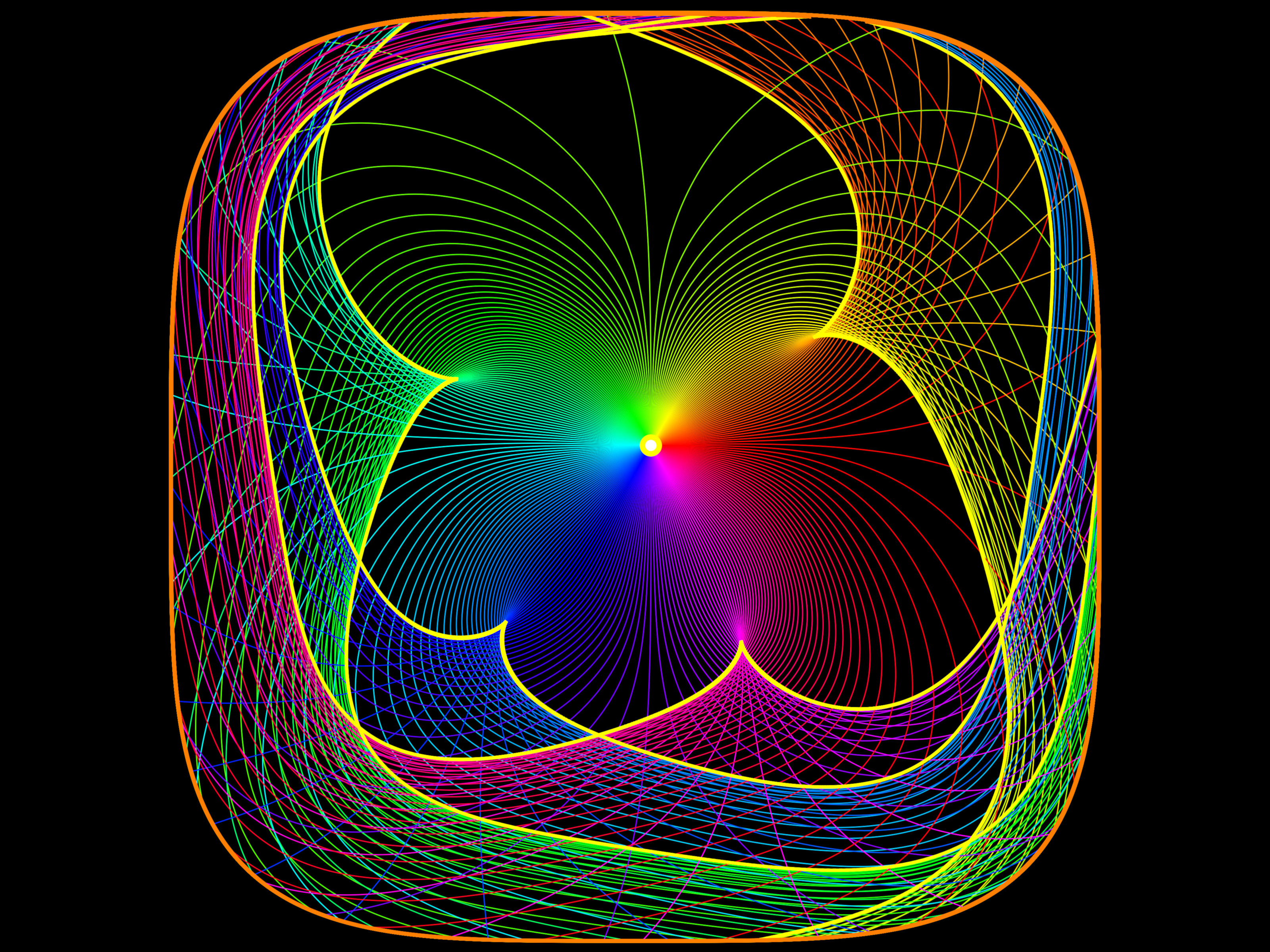The picture shows some geodesics on a surface S { z = f(x,y) = x4+y4 }. It illustrates the map expx from a disk D={x2+y2 ≤ 10 } in the tangent space TxM at the point x=(0.1,0.2,f(x,y)) in S. The program has computed the caustic (yellow) by integrating up the Jacobi field equations J'(t) = - K(r(t)) J(t) along geodesics r(t). The function K(r(t)) is the curvature. It is positive everywhere on S in this case even so the manifold is non-compact (its soul is a point). There were 24'000 geodesic paths drawn and 240 are shown. The curve r(t) hits the caustic at the point r(t) if J(t)=0.